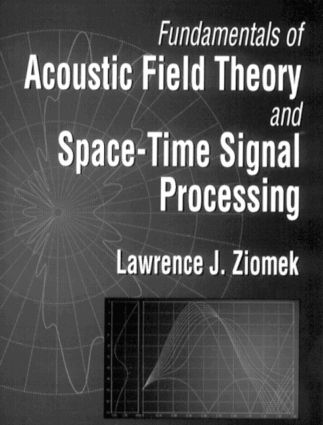# Fundamentals of Acoustic Field Theory and Space-Time Signal Processing

## 1st Edition

CRC Press

720 pages

##### Purchasing Options:\$ = USD
Hardback: 9780849394553
pub: 1994-12-28
\$205.00
x

FREE Standard Shipping!

### Description

Providing a wealth of information on fundamental topics in the areas of linear air and underwater acoustics, as well as space-time signal processing, this book provides real-world design and analysis equations. As a consequence of the interdisciplinary nature of air and underwater acoustics, the book is divided into two parts: Acoustic Field Theory and Space-Time Signal Processing. It covers the fundamentals of acoustic wave propagation as well as the fundamentals of aperture theory, array theory, and signal processing. Starting with principles and using a consistent, mainly standard notation, this book develops, in detail, basic results that are useful in a variety of air and underwater acoustic applications. Numerous figures, examples, and problems are included.

Part I

ACOUSTIC FIELD THEORY: The Linear Wave Equation and Fundamental Acoustical Quantities: Equations of Motion. Equation of Continuity. Equation of State and the Speed of Sound. Derivation of the Linear Wave Equation. Time-Average Intensity Vector and Time-Average Power. Sound-Pressure Level, Source Level, Transmission Loss, and Sound-Intensity Level. Problems. Appendices. Bibliography. Wave Propagation in the Rectangular Coordinate System: Solution of the Linear Three-Dimensional Homogeneous Wave Equation. Free-Space Propagation. Reflection and Transmission Coefficients. The Rectangular Cavity. Waveguide with Rectangular Cross-Sectional Area. The Time-Independent Free-Space Green's Function. Solution of the Linear Three-Dimensional Inhomogeneous Wave Equation with Arbitrary Source Distribution. Integral Representations of the Time-Independent Free-Space Green's Function in Rectangular Coordinates. Problems. Appendices. Bibliography. Wave Propagation in the Cylindrical Coordinate System: Solution of the Linear Three-Dimensional Homogeneous Wave Equation. Free-Space Propagation. The Cylindrical Cavity. Waveguide with Circular Cross-Sectional Area. Scattering by a Cylinder. Integral Representations of the Time-Independent Free-Space Green's Function in Cylindrical Coordinates. Reflection and Transmission of Spherical Waves at Planar Boundaries. Waveguide Models of the Ocean: Normal Modes. Waveguide Model of the Ocean: Full-Wave Solution. Problems. Appendices. Bibliography. Wave Propagation in the Spherical Coordinate System: Solution of the Linear Three-Dimensional Homogeneous Wave Equation. Free-Space Propagation. The Spherical Cavity. Scattering by a Sphere. Problems. Bibliography. Wave Propagation in Inhomogeneous Media: The WKB Approximation. Ray Acoustics. The Parabolic Equation Approximation. Problems. Appendices. Bibliography.

Part II

SPACE-TIME SIGNAL PROCESSING: Complex Aperture Theory: Coupling Transmitted and Received Electrical Signals to the Fluid Medium. Near-Field and Far-Field Directivity Functions of Volume Apertures. Linear Apertures and Far-Field Directivity Functions. Linear Apertures and Near-Field Directivity Functions. Planar Apertures and Far-Field Directivity Functions. Planar Apertures and Near-Field Directivity Functions. Directivity Index. Problems. Appendix. Bibliography. Array Theory: Linear Arrays and Far-Field Directivity Functions. Linear Arrays and Near-Field Directivity Functions. Array Gain. Planar Arrays and Far-Field Directivity Functions. Planar Arrays and Near-Field Directivity Functions. Volume Arrays and Far-Field Directivity Functions. Problems. Bibliography. Signal Processing: FFT Beamforming for Planar Arrays. Complex Envelopes. The Auto-Ambiguity Function. Time Compression/Stretch Factor, Time Delay, and Doppler Shift Expressions. Problems. Appendix. Bibliography. Fundamentals of Linear, Time-Variant, Space-Variant Filters and the Propagation of Small-Amplitude Acoustic Signals: Impulse Response and Transfer Function. Bifrequency Function. Output Frequency and Angular Spectrum. Coupling Equations and Pulse Propagation. Bibliography.

SYMBOLS AND ABBREVIATIONS.

INDEX.

### Subject Categories

##### BISAC Subject Codes/Headings:
TEC007000
TECHNOLOGY & ENGINEERING / Electrical